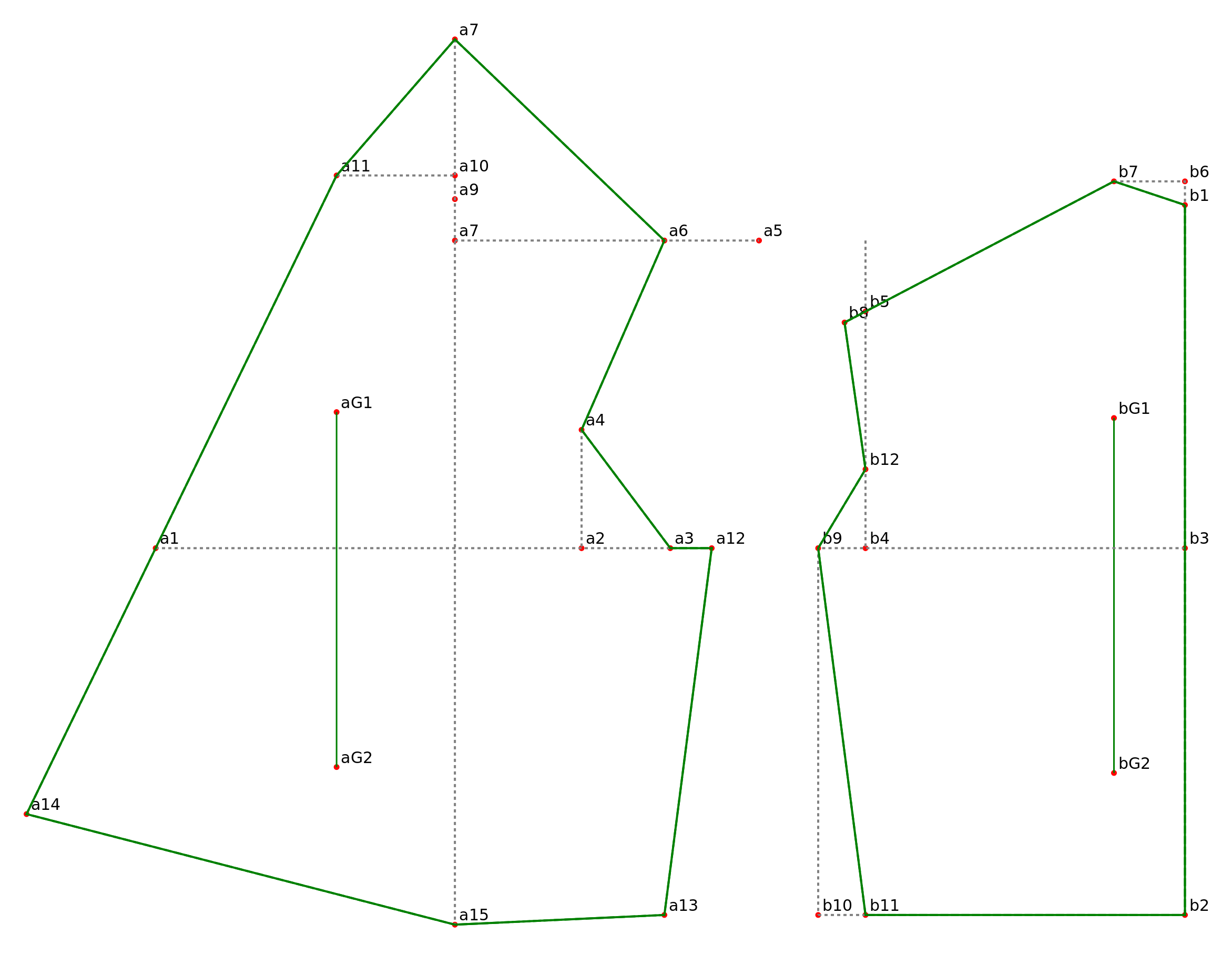# Creating a Pattern as an Inkscape Extension: Part 3

Continuation of  Part 2

STEP 6: CREATE THE SVG OBJECTS

The formulas we’ve written calculate the x,y coordinates for each point. They don’t create the SVG elements that we see on the Inkscape canvas. If we wrap each of these formulas with a function that creates an SVG element, then we calculate the pattern point coordinates and create the SVG element in one step. The functions patternPoint(parent,id,point) and patternPointXY(parent,id,x,y) create small red circles.  The parent is the pattern piece where the pattern point belongs . A is the front pattern piece, B is the back pattern piece. The SVG id should be the same as the python variable name so that there is a one-to-one mapping from the python variables to the SVG elements.  It is the SVG id not the python variable name that is displayed next to each pattern point.

b1=patternPointXY(B,’b1′,0,0)
b2=patternPoint(B,’b2′,downPoint(b1, front_waist_length))
b3=patternPoint(B,’b3′,upPoint(b2, side))
a1=patternPoint(A,’a1′,leftPoint(b3, bust_circumference/2.0))
b4=patternPoint(B,’b4′,leftPoint(b3, across_back/2.0))
b5=patternPoint(B,’b5′,upPoint(b4, armscye_circumference/3.0))
b6=patternPoint(B,’b6′,upPoint(b1, 0.5*IN))
b7=patternPoint(B,’b7′,leftPoint(b6,1.5*IN))
b8=patternPoint(B,’b8′,intersectLineAtLength(b5,b7, -0.5*IN))
a2=patternPoint(A,’a2′,leftPoint(b4, armscye_circumference/4.0))
a3=patternPoint(A,’a3′,midPoint(a2,b4))
a4=patternPoint(A,’a4′,upPoint(a2.x, 2.5*IN))
a5=patternPoint(A,’a5′,upPoint(b5.x, 1.5*IN))
a6=patternPoint(A,’a6′,leftPoint(a5, 2*IN))
a7=patternPoint(A,’a7′,leftPoint(a5, distance(b7,b8)))
a8=patternPointXY(A,’a7′,a7.x, b3.y-upper_front_height)
a9=patternPoint(A,’a9′,downPoint(a8, neck_circumference/4.0))
a10=patternPoint(A,’a10′,upPoint(a9, 0.5*IN))
a11=patternPoint(A,’a11′,leftPoint(a10, (neck_circumference/6.0)+0.25*IN ))
b9=patternPoint(B,’b9′,leftPoint(b4, 1*IN))
a12=patternPoint(A,’a12′,b9)
b10=patternPoint(B,’b10′,downPoint(b9, side))
b11=patternPoint(B ,’b11′,rightPoint(b10, 1*IN))
a13=patternPoint(A,’a13′,leftPoint(b10, 1*IN))
a14=patternPoint(A,’a14′,intersectLineAtLength(a11, a1, front_waist_length))
a15=patternPoint(A,’a15′,downPoint(a8, distance(a8, a14)))
b12=patternPoint(B,’b12′,downPoint(b5, (2/3.0)*distance(b5, b4)))
curve_a3b12=pointList(a3, b12.c1, b12.c2, b12)
b13=patternPoint(B,’b13′,intersectLineCurve(b9,b10,curve_a3b12))
a15=patternPoint(A,’a15′,b13)

STEP 7: PUT THE FORMULAS INTO A PYTHON FILE

Download  shirt_waist_allington_1.inx, shirt_waist_allington_1.py, and sewing_patterns.py and save to your inkscape user extension directory.  For Linux, this directory is /\$HOME/.config/inkscape/extensions.  For Windows it’s C:\Program Files\Inkscape\extensions. For MAC it’s ~/.config/inkscape/extensions.

Open the shirt_waist_allington_1.py file.  All of the measurements are converted to pixels with MEASUREMENT_CONVERSION:

#convert measurements
front_waist_length=self.options.m_front_waist_length*MEASUREMENT_CONVERSION
neck_circumfrence=self.options.m_neck_circumfrence*MEASUREMENT_CONVERSION
bust_circumference=self.options.m_bust_circumference*MEASUREMENT_CONVERSION
waist_circumference=self.options.m_waist_circumference*MEASUREMENT_CONVERSION
armscye_circumference=self.options.m_armscye_circumference*MEASUREMENT_CONVERSION
across_back=self.options.m_across_back*MEASUREMENT_CONVERSION
side=self.options.m_side*MEASUREMENT_CONVERSION
upper_front_height=self.options.m_upper_front_height*MEASUREMENT_CONVERSION

All of the formulas have been pasted in the section labeled #pattern points. The formulas for curve_a3b12, b13, and a15 have been commented out. The path for the front bodice starts and ends at a11. We’re checking the point formulas, so there are no curves, only Moveto and Line commands. Here is the command that creates the path string used for the seamline and cuttingline paths for the front bodice A:

path_str=formatPath(‘M’,a11,’L’,a14,’L’,a15,’L’,a13,’L’,a12,’L’,a3,’L’,a4,’L’,a6,’L’,a8,’L’,a11)